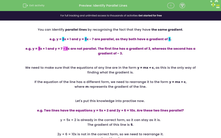# Identify Parallel Lines

In this worksheet, students will identify if lines are parallel by comparing gradients represented by m in the equation y = mx + c.Key stage:  KS 4

Year:  GCSE

GCSE Subjects:   Maths

GCSE Boards:   Pearson Edexcel, OCR, Eduqas, AQA,

Curriculum topic:   Algebra, Graphs of Equations and Functions

Curriculum subtopic:   Graphs Straight Line Graphs

Difficulty level:#### Worksheet Overview

You can identify parallel lines by recognising the fact that they have the same gradient.

e.g. y = 3x + 1 and y = 3x - 7 are parallel, as they both have a gradient of 3.

e.g. y = 3x + 1 and y = 7 - 3x are not parallel. The first line has a gradient of 3, whereas the second has a gradient of - 3.

We need to make sure that the equations of any line are in the form y = mx + c, as this is the only way of finding what the gradient is.

If the equation of the line has a different form, we need to rearrange it to the form y = mx + c, where m represents the gradient of the line.

Let's put this knowledge into practise now.

e.g. Two lines have the equations y = 5x + 2 and 2y + 6 = 10x. Are these two lines parallel?

y = 5x + 2 is already in the correct form, so it can stay as it is.

The gradient of this line is 5.

2y + 6 = 10x is not in the correct form, so we need to rearrange it.

2y = 10x - 6

y = 5x - 3

The gradient of the second line is 5.

As the gradients of both lines are 5, they are parallel.

In this activity, we will identify if lines are parallel by comparing gradients represented by m in the equation y = mx + c.

### What is EdPlace?

We're your National Curriculum aligned online education content provider helping each child succeed in English, maths and science from year 1 to GCSE. With an EdPlace account you’ll be able to track and measure progress, helping each child achieve their best. We build confidence and attainment by personalising each child’s learning at a level that suits them.

Get started